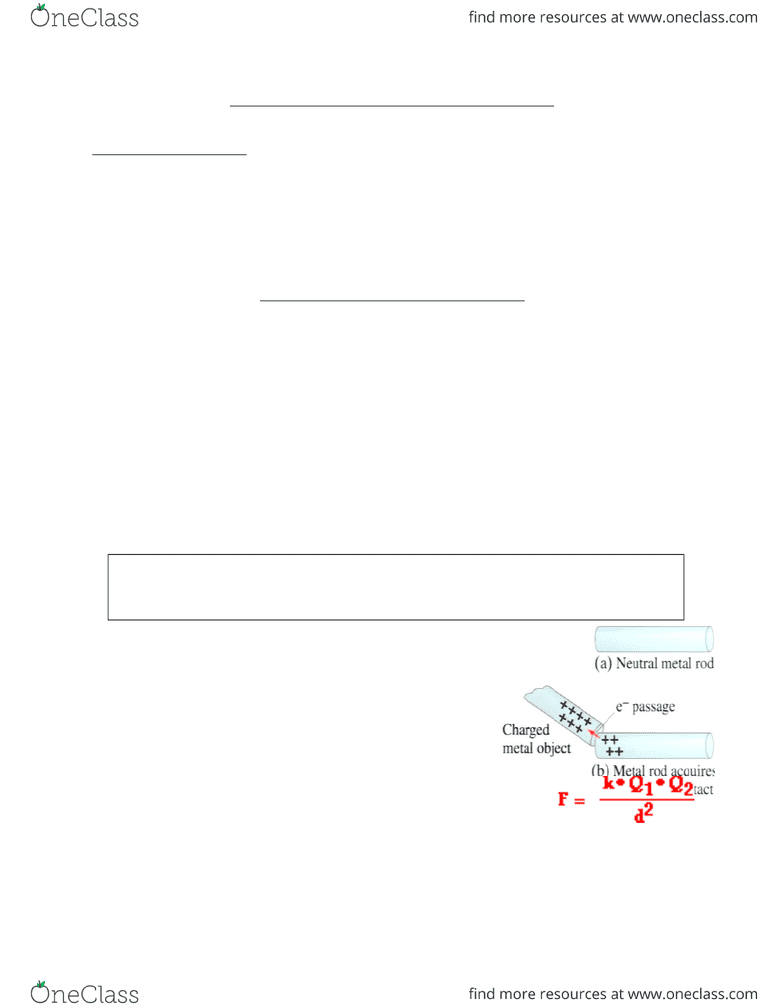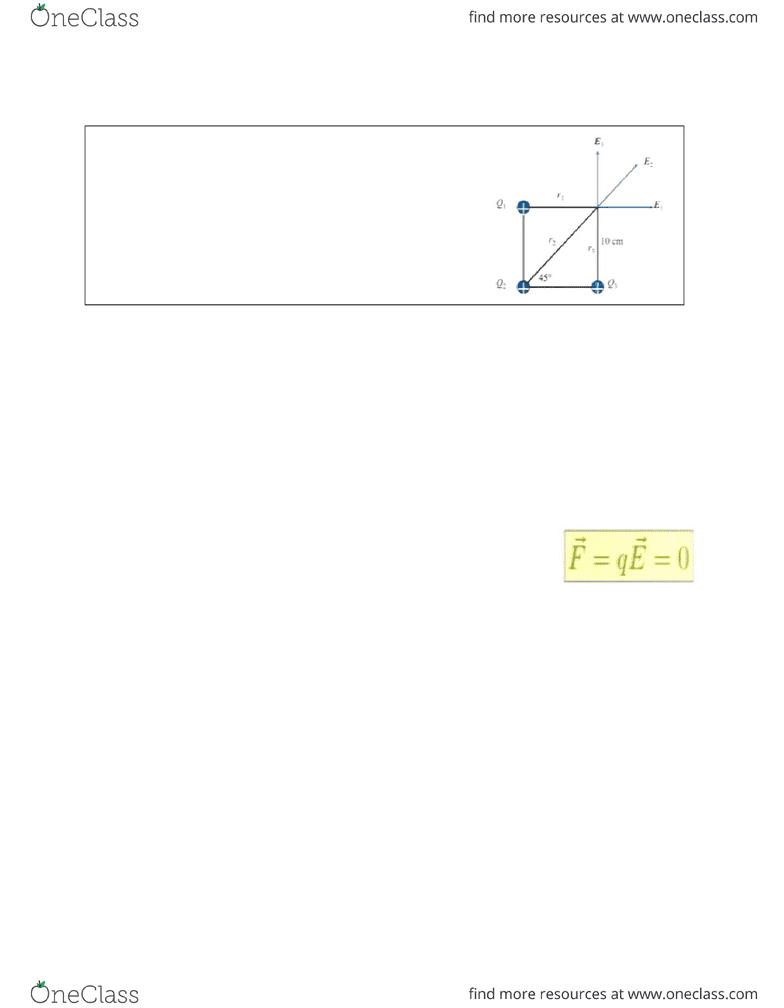Study Guides (380,000)
CA (150,000)
McGill (6,000)
Physics (100)
Midterm

# PHYS 102 Study Guide - Midterm Guide: Electric Potential Energy, R V R, Electric Field

Department
Physics
Course Code
PHYS 102
Professor
Nick Provatas
Study Guide
Midterm

This preview shows pages 1-2. to view the full 6 pages of the document.Fundamental Concepts for Midterm of PHYS 102
Midterm: (20%) Topics:
Electric Charge & Electric Field (Ch. 16)
Electric Potential & Potential Energy (Ch. 17)
Electric Current (Ch.18)
D.C. Circuits (Ch.19 – up to & including 19.2)
Electric Charge & Electric Field (Ch. 16)
Static Electricity: Electric Charge & its Conservation
static electricity: object charged due to rubbing
2 types of electric charge: attraction or repulsion (+ve of –ve)
Law of conservation of electric charge: the net amount of electric charge produced in
any process is 0, no charge is created nor destroyed
Charging by Induction in Metals & Insulators
Conductor allows charges to move freely in it
induction happens only when the charged object are literally so close to contacting the
insulating object in insulating objects (induced charge separation)
conductors: free charges, bringing a charge near causes a polarization which causes
attraction, but if there is already a charge on the conductor, you cannot tell (situation
dependent)
Conceptual question: what happens if the metal sphere is originally positive, and bring a
negative near? There will be attraction; but if sphere is originally negative, you cannot tell
(depends on charges and distances related to coulomb’s law, which will determine the
magnitude of the force)
Charge Transfer in Conductors
Charging conducting (metal) objects: induction causes separation and transfer of
charges in contacting metals by conduction
Coulomb’s Law
Coulomb’s law: the electrical force between two charged objects is directly proportional
to the product of the quantity of charge on the objects and inversely proportional to the
square of the separation distance between the two objects
gives the *magnitude* of the force
the magnitude is always a positive quantity (do not use the signs on the charges in this
equation when calculating the magnitude of the force)
the direction of the force is determined from either the charges of the same sign repel
each other, or the charges with opposite signs attract each other
Electric Fields
electric field is a property of the space around a charge, whereas how force talks about
how 2 charges attract each other

Only pages 1-2 are available for preview. Some parts have been intentionally blurred.electric fields add like vectors (force and electric fields are vector quantities)
Example Question:
What is x component of E2? kQ2/r22 *cos(45)
What is E total in x direction?
Etotal in x direction = kQ1/r12 + kQ2/r22 *cos(45)
What is Etotal in y direction?
Etotal in x direction = kQ3/r32 + kQ2/r22 *sin(45)
Electric Field Lines
they are a graphical representation of electric fields
they point in the direction of a positive unit charge
electric field vector is tangent to the electric field line
electric field lines start from a positive charge and end on a negative charge (or start and
end at infinity)
the number of the electric field lines originating from a charge q is proportional to q
the number of the electric field lines per unit area is proportional to the magnitude of the
electric field
electric field lines do not cross
Electric Field Lines Inside & Outside Conductors
in charged conductors, charges are free to move, making the electric field inside a
charged conductor 0
when you’re inside a conductor there is no electric field (therefore the potential is all the
same value, because to have an electric field, you need to have a change in potential,
so the potential is a constant)
in charged conductors, the only possible way this can happen if the net charges reside
on its surface
electric field is perpendicular to the surface
Electric Potential & Potential Energy (Ch. 17)
Electric Potential & Potential Energy
Electrical potential energy: negative work done by electric field during the displacement
of a charge q from point A to B
Work is a force applied over a distance (mechanical work)
###### You're Reading a Preview

Unlock to view full version